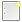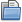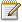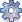Direction: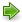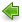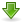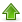Mode: Code String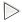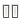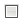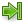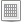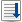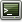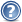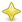# jsFunge IDE

Grid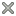StackTerminalInputEnter a char:
InputEnter an integer:
ImportEnter the program to import:
Help## What is the Befunge 93 ?

Befunge is a stack-based, reflective, esoteric programming language. It differs from conventional languages in that programs are arranged on a two-dimensional grid. "Arrow" instructions direct the control flow to the left, right, up or down, and loops are constructed by sending the control flow in a cycle.

## Instruction set

### Flow direction:

• >  Start moving right
• <  Start moving left
• v  Start moving down
• ^  Start moving up
• ?  Start moving in a random direction

### Matematical and logical operations:

• +  Addition: pop a and b, and then push a+b
• -  Subtraction: pop a and b, and then push b-a
• *  Multiplication: pop a and b, and then push a*b
• /  Division (integer): pop a and b, and then push b/a
• %  Modulo: pop a and b, and then push b%a
[FIXME: if a=0, ask the user for the result]
• !  Logical NOT: pop a value. If the value is zero,
push 1; otherwise, push zero.

### Tests:

• `  Greater than: pop a and b, then push 1 if b>a,
otherwise push zero.

### Jumps:

• _  Pop a value; move right if value=0, left otherwise.
• |  Pop a value; move down if value=0, up otherwise.
• #  Skip the next cell

### Stack operations:

• 0-9  Push the number on the stack
•   :  Duplicate value on top of the stack
•   \$  Pop a value from the stack
•   \  Swap two values on top of the stack.

### Input / Output:

• .  Pop value and output as an integer.
• ,  Pop value and output as an ASCII character.
• p  Put: pop y, x and v, then change the character at
the position (x,y) in the program to the character
with ASCII value v. [FIXME: Out of range]
• g  Get: pop y and x, then push ASCII value of the
character at that position in the program
[FIXME: Out of range]
• &  TODO (Not yet implemented).
• ~  TODO (Not yet implemented).

### Other instructions:

• "  Start string mode: push each character's ASCII
value all the way up to the next ".
• @  The end of the program.

About jsFunge IDE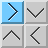## jsFunge IDE 0.1

A Befunge 93 Editor, Interpreter and Debugger